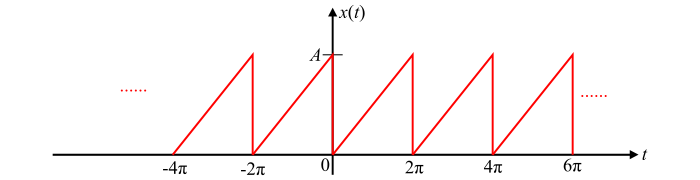# Fourier Cosine Series â€“ Explanation and Examples

The cosine form of Fourier series is the alternate form of the trigonometric Fourier series. The cosine form Fourier series is also known as polar form Fourier series or harmonic form Fourier series.

The trigonometric Fourier series of a function x(t) contains sine and cosine terms of the same frequency. That is,

$$\mathrm{x(t)=a_{0}+\sum_{n=1}^{\infty}a_{n}\:cos\:n\omega_{0} t+b_{n}\:sin\:n\omega_{0} t… (1)}$$

Where,

$$\mathrm{a_{0}=\frac{1}{T}\int_{t_{0}}^{(t_{0}+T)}x(t)\:dt}$$

$$\mathrm{a_{n}=\frac{2}{T}\int_{t_{0}}^{(t_{0}+T)}x(t)\:cos\:n\omega_{0} t\:dt}$$

$$\mathrm{b_{n}=\frac{2}{T}\int_{t_{0}}^{(t_{0}+T)}x(t)\:sin\:n\omega_{0} t\:dt}$$

In equation (1), by multiplying the numerator and denominator of the sine and cosine terms with ($\sqrt{a_{n}^{2}+b_{n}^{2}}$), we get,

$$\mathrm{x(t)=a_{0}+\sum_{n=1}^{\infty}\left( \sqrt{a_{n}^{2}+b_{n}^{2}}\right)\left( \frac{a_{n}}{\sqrt{a_{n}^{2}+b_{n}^{2}}}cos\:n\omega_{0} t+\frac{b_{n}}{\sqrt{a_{n}^{2}+b_{n}^{2}}}sin\:n\omega_{0} t\right)… (2)}$$

Putting the values in the equation (2) as,

$$\mathrm{a_{0}=A_{0}}$$

$$\mathrm{\sqrt{a_{n}^{2}+b_{n}^{2}}=A_{n}… (3)}$$

$$\mathrm{\frac{a_{n}}{\sqrt{a_{n}^{2}+b_{n}^{2}}}=cos\:\theta_{n}\:\:and\:\:\frac{b_{n}}{\sqrt{a_{n}^{2}+b_{n}^{2}}}=-sin\:\theta_{n}}$$

We obtain,

$$\mathrm{x(t)=A_{0}+\sum_{n=1}^{\infty}A_{n}(cos\:\theta_{n}\:cos\:n\omega_{0} t-sin\:\theta_{n}\:sin\:n\omega_{0} t)}$$

$$\mathrm{\Rightarrow\:x(t)=A_{0}+\sum_{n=1}^{\infty}A_{n}\:cos(n\omega_{0} t+\theta_{n})… (4)}$$

Where,

$$\mathrm{\theta_{n}=-tan^{-1} \left(\frac{b_{n}}{a_{n}}\right)… (5)}$$

The expression in equation (4) is the cosine representation of the function x(t) which contains sinusoids of frequencies $\omega_{0},2\omega_{0},3\omega_{0},...$. In this expression, $A_{0}$is called the DC component and the term $A_{n}[cos(n\omega_{0} t+\theta_{n})]$ is called the nth harmonic component of the function x(t).

Here, $A_{n}$ represents the harmonic amplitude or amplitude coefficient or spectral amplitude of the cosine Fourier series, and $\theta_{n}$ is called the phase angle or phase coefficient or spectral phase of the cosine Fourier series.

## Numerical Example

Find the cosine Fourier series for the waveform shown in the figure.Solution

The waveform of the figure can be described mathematically as follows −

$$\mathrm{x(t)=\frac{A}{2\pi}t;\:\:for\:0 ≤\:t\:≤2\pi}$$

Let

$$\mathrm{t_{0}=0\:\:and\:\:(t_{0}+T)=2\pi}$$

Therefore, the fundamental frequency of the given function is,

$$\mathrm{\omega_{0}=\frac{2\pi}{T}=\frac{2\pi}{2\pi}=1}$$

Now, the coefficients of the cosine Fourier series can be determined as follows −

$$\mathrm{A_{0}=a_{0}=\frac{1}{T}\int_{t_{0}}^{(t_{0}+T)}x(t)\:dt}$$

$$\mathrm{\Rightarrow\:A_{0}=\frac{1}{2\pi}\int_{0}^{2\pi}\frac{A}{2\pi}t\:dt=\frac{A}{(2\pi)^{2}}\left [\frac{t^{2}}{2} \right ]_{0}^{2\pi}=\frac{A}{(2)}}$$

$$\mathrm{\therefore\:A_{0}=\frac{A}{2}}$$

Again,

$$\mathrm{a_{n}=\frac{2}{T}\int_{t_{0}}^{(t_{0}+T)}x(t)\:cos\:n\omega_{0} t\:dt}$$

$$\mathrm{\Rightarrow\:a_{n}=\frac{2}{2\pi}\int_{0}^{2\pi}(\frac{A}{2\pi}t)cos\:nt\:dt=\frac{2A}{(2\pi)^{2}}\int_{0}^{2\pi}t\:cos\:nt\:dt}$$

On solving the above integration, we get,

$$\mathrm{a_{n}=\frac{2A}{(2\pi)^{2}}=0}$$

Also,

$$\mathrm{b_{n}=\frac{2}{T}\int_{t_{0}}^{(t_{0}+T)}x(t)sin\:n\omega_{0} t\:dt=\frac{2}{2\pi}\int_{0}^{2\pi}(\frac{A}{2\pi}t)sin\:nt\:dt}$$

$$\mathrm{\Rightarrow\:b_{n}=\frac{2A}{(2\pi)^{2}}\int_{0}^{2\pi}t\:sin\:nt\:dt}$$

On solving the above integration, we obtain,

$$\mathrm{b_{n}=\frac{2A}{(2\pi)^{2}}(\frac{-2\pi}{n})=\frac{-A}{n\pi}}$$

Hence, amplitude coefficient of the cosine Fourier series is,

$$\mathrm{A_{n}=\sqrt{a_{n}^{2}+b_{n}^{2}}=\sqrt{0+\left(\frac{-A}{n\pi}\right)^{2}}=\frac{A}{n\pi}}$$

And the phase coefficient of the cosine Fourier series is,

$$\mathrm{\theta_{n}=-tan^{-1}\left(\frac{b_{n}}{a_{n}}\right)=-tan^{-1}\left[\frac{\left(\frac{-A}{n\pi}\right)}{0} \right]=-\frac{\pi}{2}}$$

Therefore, the cosine Fourier series of the waveform is,

$$\mathrm{x(t)=A_{0}+\sum_{n=1}^{\infty}A_{n}\:cos(n\omega_{0} t+\theta_{n})}$$

$$\mathrm{\Rightarrow\:x(t)=\frac{A}{2}+\sum_{n=1}^{\infty}\frac{A}{n\pi}cos(nt-\frac{\pi}{2})… (6)}$$

The expression in equation (6) is the cosine Fourier series representation of the given waveform.